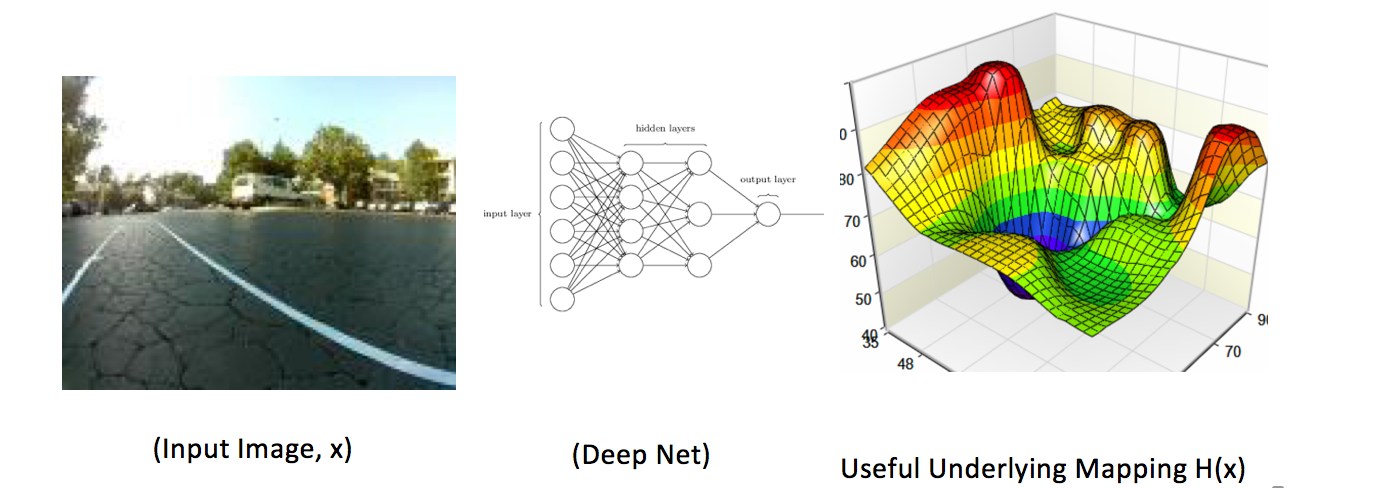# Yet Another ResNet Tutorial (or not)

The purpose of this article is to expose the most fundamental concept driving the design and success of ResNet architectures. Many blogs and articles go on and on describing how this architecture is laid out, and show images of large networks as well as apprehensive accuracy tables and loss plots. I hope to excuse myself from reiterating the obvious, and hopefully really cutting to the kernel.

The ResNet architecture is often touted as the state-of-the-art in image classification tasks. The ability to construct arbitrarily deep layers, followed by the popular ‘we need to go deeper’ heuristics makes ResNet the prodigy of deep learning architectures. The constituent building unit of the ResNet architecture is the ResNet block, as shown below:

The key idea driving the design of ResNet is actually best described in the original paper itself rather than in any other blogs I’ve seen:

“So rather than expect stacked layers approximate H(x) (the underlying mapping function), we explicitly let these layers approximate a residual function F(x) := H(x) -x. The original function thus becomes F(x) + x. ”

If you’re like me, you’re trying to wrap your head around this and wondering what the hell’s going on here... What is the original mapping function, (F(x)), H(x), and why the residual? The original paper goes into a lot of detail, and between the residual function, the degradation diagnosis, and other technical jargon, the heart of the matter is sometimes lost in a casual read.

You see.. a deep neural net has a specific task, and that is: Given an x, the net’s one and only goal is to find an underlying useful mapping function H(x). The function H(x) is useful because it attempts to classify the given image into specified class labels, sub-segments, captions, or whatever the output it is you’re trying to produce. So let’s visualize it as this:Our Deep Net needs to find the mapping function H(x)

The residual way of thinking is to say: You know what.. let’s not try to map it to the mapping function H(x). Instead, let me try to map it to a different function F(x)!

Wait.. what is F(x)? This is where all the knowledge and intuition kicks in. The authors of ResNet do not want the net to try approximate just any random F(x). Instead, they want it (the net) to approximate a very well-defined, albeit simple, function F(x), such that F(x) := H(x) -x. This function is what the authors call by the name: “residual function”.

Now, here’s the twist: Our good-old pal H(x), the original mapping function, is still the only function that we really (truly) love and need! So, in order to fool our deep-learning net to find the function H(x), we actually modify the input so that instead of supplying the net with the original input: x, we actually feed it F(x)+x!

And there you have it: this is how the residual block and how the skip-connections work. Remember that the skip connection allows for an input channel which is F(x)+x, but what we’re really wanting to find is the (one-and-only) function H(x). At the heart of the matter, this is all there it is.

Original Reference Paper for ResNet:

https://arxiv.org/abs/1512.03385